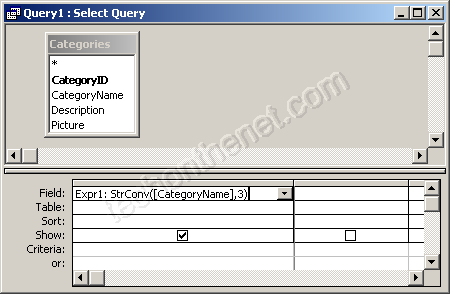## 8/01/2012

### Capitalize each word in a string (similar to InitCap in Oracle)

In Access, the StrConv function returns a string converted as specified.
The syntax for the StrConv function is:
StrConv ( text, conversion, LCID )
text is the string that you wish to convert.

conversion is the type of conversion to perform. The following is a list of valid parameters for conversion.
Parameter Value Description
vbUpperCase 1 Converts the string to all uppercase.
vbLowerCase 2 Converts the string to all lowercase.
vbProperCase 3 Converts the first letter to every word to uppercase. All other characters are left as lowercase. This option is similar to the InitCap function in Oracle.
vbUnicode 64 Converts the string to Unicode.
vbFromUnicode 128 Converts the string from Unicode to the default code page of the system.
Note: If you are using the StrConv function in VBA code, you can use the vb parameter listed in the table above. If you are using this function in a query, you will have to use the numeric value.
LCID is optional. If this parameter is omitted, the StrConv function assumes the system LocaleID.

## For Example:

 StrConv ("tech on the net", 1) would return "TECH ON THE NET" StrConv ("TECH ON THE NET", 2) would return "tech on the net" StrConv ("TECH ON THE NET", 3) would return "Tech On The Net"

## VBA Code:

The StrConv function can be used in VBA code. For example:
Dim LResult As String
LResult = StrConv ("TECH ON THE NET", 3)
In this example, the variable called LResult would now contain the value "Tech On The Net".

Since we are using the StrConv function in VBA, we could also use the vb values for the conversion parameter, such as:
Dim LResult As String
LResult = StrConv ("TECH ON THE NET", vbProperCase)
In this example, the variable LResult would also contain the value "Tech On The Net".

## SQL/Queries:

You can also use the StrConv function in a query.